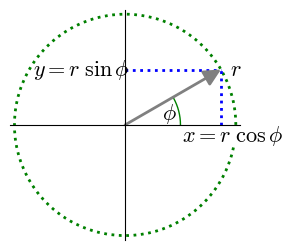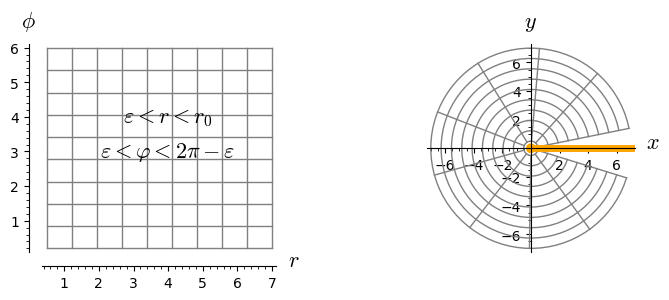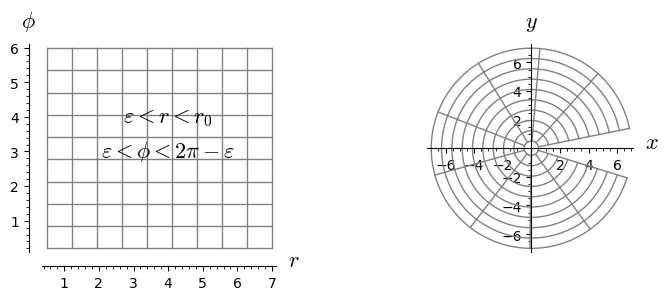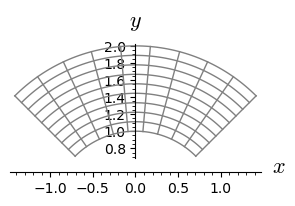# 2. Examples of charts. Cartesian and spherical coordinates¶

This notebook is part of the Introduction to differentiable manifolds in SageMath by Andrzej Chrzeszczyk (Jan Kochanowski University of Kielce, Poland).

In :
version()

Out:
'SageMath version 9.6, Release Date: 2022-05-15'

In every example of a manifold, some charts must be defined, so in the next four notebooks we present examples of such definitions.

In the present notebook we are using the EuclideanSpace module, since some coordinate systems are predefined in this special case.

Generally, in the Manifold module, the charts (or coordinate systems) and the transition maps must be defined by the user.

### 2-dimensional case¶

Example 2.1

First consider the case of 2-dimensional Euclidean space.

In :
E.<x,y> = EuclideanSpace()  # number of variables determines dimension
cartesian.<x,y> = E.cartesian_coordinates()  # Cartesian coordinates
polar.<r,ph> = E.polar_coordinates()         # polar coordinates


Let us check, that the domains of these maps are whole Euclidean space $E^2$.

In :
cartesian.domain(), polar.domain() # domains of Cart.and polar coord.

Out:
(Euclidean plane E^2, Euclidean plane E^2)

Now let us check the ranges of the coordinate variables.

In :
print(cartesian.coord_range())           # Cartesian coord. ranges
print(polar.coord_range())               # polar coord. ranges

x: (-oo, +oo); y: (-oo, +oo)
r: (0, +oo); ph: [0, 2*pi] (periodic)


The result: $r\in (0,+\infty),\ \ \phi\in [0,2\pi]$ (periodic) shows that the predefined polar coordinates do not define a one-to one map onto an open subset of $R^2$ (the domain and the range of polar coordinates will be restricted below).

The transition from the polar coordinate system to the Cartesian coordinate system is given by $x=r\cos\varphi,\ \ y=r\sin\varphi$:

In :
polar_to_cart = E.coord_change(polar,cartesian)  # polar -> Cartesian
polar_to_cart.display()                        # transition

Out:
x = r*cos(ph)
y = r*sin(ph)
In :
po1 = {'thickness': 2, 'linestyle': ':'}            # lines parameters
po2 = {'fontsize': 16, 'color': 'black'}            # text parameters
t = var('t')                                     # symbolic variable
p0 = parametric_plot((0.5*cos(t), 0.5*sin(t)),
(t,0,pi/6), color='green')                # circular arc
p1 = parametric_plot((cos(t),sin(t)),
(t,0,2*pi-0.2), color='green', **po1)       # dotted circle
p2 = parametric_plot((cos(pi/6),t),
(t,0,sin(pi/6)), color='blue', **po1)       # vertical dot-line
p3 = parametric_plot((t,sin(pi/6)),
(t,0,cos(pi/6)), color='blue', **po1)       # horizontal dot-line
a = arrow((0,0), (cos(pi/6),sin(pi/6)), color='grey')      # arrow
t0 = text("$y=r\\; \\sin\\phi$", (-0.4,sin(pi/6)), **po2)     # y=rsin(phi)
t1 = text("$x=r\\;\\cos\\phi$", (cos(pi/6)+0.1,-0.1), **po2)  # x=rcos(phi)
t2 = text("$r$", (1,0.5), **po2)                            # r
t3 = text("$\\phi$", (0.4,0.1), **po2)                      # phi
(p0+p1+p2+p3+t0+t1+t2+t3+a).show(ticks=[[],[]], figsize=[3,3])The polar coordinates defined on the entire $E^2$ are not homeomorphic. They do not define a one-to-one mapping of $E^2$ onto an open subset of $R^2$, since points of the form $(r, \varphi)$ and $(r, \varphi+2\pi)$ pass to the same point.

To obtain homeomorphic charts on an open set and smooth invertible transitions we can restrict ourselves to the open subset of $E^2$ with half line {y=0, x>=0} excluded.

With this restriction in mind the inverse transition is well defined.

In :
cart_to_polar = E.coord_change(cartesian, polar) # transition
cart_to_polar.display()                          # Cartesian -> polar

Out:
r = sqrt(x^2 + y^2)
ph = arctan2(y, x)

The nonvanishing of the Jacobian determinants of the one-to-one smooth mapping $f$ at all points of its domain means that the mapping $f^{-1}$ inverse to $f$ is smooth. This follows from the local inverse function theorem. So let us check the Jacobians of the transition maps.

In :
print(polar_to_cart.jacobian_det())   # det(Jacobian(polar->Cart))
print(cart_to_polar.jacobian_det())   # det(Jacobian(Cart->polar))

r
1/sqrt(x^2 + y^2)


This shows (again) that the point (0,0) should be excluded from the domain of transition maps.

As was mentioned above, to obtain homeomorphic charts on an open set and smooth invertible transitions we can restrict ourselves to the subset of $E^2$ with half line $\{y=0, x\geq 0\}$ excluded.

In the definition of the open set $U$, below we show how to make the corresponding restrictions. The round brackets in (y!=0, x<0) denote the inclusive OR (true if at least one of two inputs is true). Thus (y!=0, x<0) is equivalent to $\ \ \neg(y=0\ \&\ x\geq 0)\$ i.e., the half line $\{y=0, x\geq 0 \}$ is excluded.

In :
# U is the open subset of E^2 with {y=0,x>=0} excluded
U = E.open_subset('U', coord_def={cartesian: (y!=0, x<0)})
polarU = U.chart(r'r:(0,+oo) ph:(0,2*pi):\phi')    # polar coord.
cartU = cartesian.restrict(U)        # Cartesian coordinates on U
polarU.coord_range()                 # ranges of polar on U

Out:
r: (0, +oo); ph: (0, 2*pi)

The command coordinate_chart.plot allows to plot coordinate lines of the chart coordinate_chart.

Example 2.2

In our next example, coordinate_chart is polar (try polar.plot? to see more information). As the first argument one can use both polar and cartesian. In polar coordinates the set $\ \ \varepsilon < r < r_0$, $\ \ \varepsilon < \varphi < 2\pi-\varepsilon\ \$ is an open rectangle. In Cartesian coordinates its image is the circle with a small sector and some small neighborhood of (0.0) excluded.

In :
# To define a set with some neighborhood of the half line {y=0, x>=0}
# excluded it is sufficient to use appropriate ranges of parameters r,ph
E.<x,y> = EuclideanSpace()  # number of variables determines dimension
cartesian.<x,y> = E.cartesian_coordinates()     # Cartesian coordinates
polar.<r,ph> = E.polar_coordinates()            # polar coordinates
p0 = polar.plot(polar, ranges={r: (0.5,7), ph: (0.2,2*pi-0.3)},
number_values=10, color='grey')  # plot coordinate lines r,ph
# in polar coordinates
t0 = text("$\\varepsilon < r < r_0$", (4, 4), fontsize=16, color='black')
s0 = text("$\\varepsilon < \\varphi < 2\\pi-\\varepsilon$", (4, 3),
fontsize=16, color='black')               # textual description
r0 = p0 + t0 + s0                                   # combine previous plots
p1 = polar.plot(cartesian, ranges={r: (0.5,7), ph: (0.2,2*pi-0.3)},
number_values=10, color='grey')         # plot r,ph lines in Cart.coord.
p2 = point2d((0,0), size=50, color='orange')   # plot excluded neighb. of (0,0)
p3 = plot(0*x,(0,7), thickness=5, color='orange') # plot excluded halfline
p = p1 + p2 + p3                                    # combine plots
graphics_array([r0,p]).show(figsize=(8,3))          # plot graphics arrayExample 2.3

The same plot with maps restricted to an open subset $U$ of $E^2$.

In :
# continuation
U = E.open_subset('U', coord_def={cartesian: (y!=0, x<0)})
polarU = U.chart(r'r:(0,+oo) ph:(0,2*pi):\phi')   # polar coord. on U
cartU = cartesian.restrict(U)                     # Cart. coord. on U
p0 = polarU.plot(polarU, ranges={r: (0.5,7), ph: (0.2,2*pi-0.3)},
number_values=10, color='grey') # plot coord.lines r,ph in polar coord.
# transition from polarU to cartU:
FU = U.continuous_map(U, {(polarU, cartU): [r*cos(ph), r*sin(ph)]}, name='FU')

p1 = polarU.plot(cartU, mapping=FU, ranges={r:(0.5,7), ph:(0.2,2*pi-0.3)},
number_values=10, color='grey') # plot the image of coord lines r,ph
t0 = text("$\\varepsilon < r < r_0$", (4, 4), fontsize=16, color='black')
s0 = text("$\\varepsilon < \\phi < 2\\pi-\\varepsilon$",
(4, 3), fontsize=16, color='black')         # textual description
r0 = p0 + t0 + s0                                     # combine plots
graphics_array([r0, p1]).show(figsize=(8,3))     # graphics arrayUsing coordinate lines one can easily plot images of any rectangle $r_0< r <r_1, \ \ \phi_0< \phi <\phi_1$:

In :
p = polar.plot(cartesian,ranges={r: (1,2), ph: (pi/4,3*pi/4)},
number_values=10, color='grey')    # plot coord.lines r,ph
p.show(figsize=3)         # in the subset r0<r<r1, ph0<ph<ph1### 3-dimensional case¶

Example 2.4

Now consider the 3-dimensional case.

In :
E.<x,y,z> = EuclideanSpace()  # number of variables determines the dimension
cartesian.<x,y,z> = E.cartesian_coordinates()  # Cartesian coordinates in E^3
spherical.<r,th,ph> = E.spherical_coordinates()  # polar coordinates in E^3


Check the domains and variables ranges.

In :
cartesian.domain(), spherical.domain()  # domains of Cart. and polar coord.

Out:
(Euclidean space E^3, Euclidean space E^3)
In :
print(cartesian.coord_range())         # ranges of Cartesian coordinates
print(spherical.coord_range())         # ranges of spherical coordinates

x: (-oo, +oo); y: (-oo, +oo); z: (-oo, +oo)
r: (0, +oo); th: (0, pi); ph: [0, 2*pi] (periodic)


As we can see, the spherical coordinates don't define a homeomorphism on the entire $E^3$ (appropriate restrictions will be given below).

The transition from the spherical coordinate system to the Cartesian coordinate system is given by the functions

$x = r\cos\phi\sin\theta,\quad y = r\sin\phi\sin\theta,\quad z = r\cos\theta$:

In :
sph_to_cart = E.coord_change(spherical, cartesian) # transition
sph_to_cart.disp()                                 # spher-> Cartesian

Out:
x = r*cos(ph)*sin(th)
y = r*sin(ph)*sin(th)
z = r*cos(th)
In :
var('u v t')                                   # symb.var.

po1 = {'thickness': 5, 'color': 'darkblue'}         # param.
po2 = {'fontsize': 20, 'color': 'black'}
po3 = {'size': 7, 'color': 'black'}

ax = line3d([(0,0,0), (1+0.15,0,0)], **po1)     # axes
ax += line3d([(0,0,0), (0,1+0.15,0)], **po1)
ax += line3d([(0,0,0), (0,0,1+0.15)], **po1)
ax += text3d("x", (1.25,0,0), **po2)
ax += text3d("y", (0,1.25,0), **po2)
ax += text3d("z", (0.,0.,1.25), **po2)
# hemisphere:
s = parametric_plot3d((cos(u)*cos(v), sin(u)*cos(v), sin(v)),
(u,0,2*pi), (v,0,pi/2), opacity=0.9, color='lightgrey')

a = 0.59                                         # triangle
tr = line3d([(0,0,0), (a,a,0), (a,a,a), (0,0,0)], **po1)

dots = point3d([(0.5*cos(t), 0.5*sin(t), 0)        # dots
for t in srange(0,pi/4,0.1)], **po3)
dots+=point3d([(0.5*cos(t), 0.5*cos(t), 0.5*sin(t))
for t in srange(pi/4,pi/2,0.1)], **po3)

t = text3d("(x,y,z)", (0.6,0.8,0.7),**po2)        # variables
t += text3d("φ", (0.7,0.3,0.0), **po2)
t += text3d("θ", (0.,0.2,0.5), **po2)
# combine plots
(ax+s+tr+dots+t).rotateZ(-pi/8).show(frame=False)


The spherical coordinates defined on the entire $E^3$ are not homeomorphic. They do not define a one-to-one mapping of $E^3$ onto an open subset of $R^3$, since for example, points of the form (𝑟,𝜃,𝜑) and (𝑟,𝜃,𝜑+2𝜋) pass to the same point.

To obtain homeomorphic charts on an open set and smooth invertible transitions, we can restrict ourselves to the open subset of $E^3$ with half plane {y=0, x>=0} excluded.

With this restriction in mind the inverse transition is well defined.

In :
cart_to_sph = E.coord_change(cartesian, spherical); # transition
cart_to_sph.display()                 # Cartesian -> spherical

Out:
r = sqrt(x^2 + y^2 + z^2)
th = arctan2(sqrt(x^2 + y^2), z)
ph = arctan2(y, x)

Let us check the determinants of Jacobians of the transition maps.

In :
sph_to_cart.jacobian_det()           # det(Jacobian(spher->cart))

Out:
r^2*sin(th)
In :
cart_to_sph.jacobian_det()           # det(Jacobian(cart->spher))

Out:
1/(sqrt(x^2 + y^2 + z^2)*sqrt(x^2 + y^2))

Example 2.5

As we have observed, to obtain well defined transitions, we can restrict ourselves to the open subset of $E^3$
with half plane {y=0, x>=0} excluded.

Below, we show how to make appropriate restriction.

In :
# continuation                      # define open subset U
U = E.open_subset('U', coord_def={cartesian: (y!=0, x<0)})
spherU.<r,th,ph> = U.chart(r'r:(0,+oo) th:(0,pi):\theta ph:(0,2*pi)')
cartU = cartesian.restrict(U)         # restrict Cart. coord to U


To make a plot with some neighborhood of the half plane {y=0, x>=0} excluded it is sufficient to use appropriate ranges of parameters.

In :
# plot coord. lines using the values 0, 5.7 of r;
#                      values 0.3, 2pi-0.3 of ph;
#          and 20 values of th from [0.3,pi-0.3];

p1 = spherical.plot(cartesian,
ranges={r: (0.5,7), th: (0.3,pi-0.3), ph: (0.3,2*pi-0.3)},
number_values={r: 2, ph: 2, th: 20},
color={r: 'red', ph: 'grey', th: 'red'},
thickness=1, label_axes=False)    # plot coord. lines r,th,ph

In :
po1 = {'thickness': 3, 'color': 'darkblue'}         # param.
po2 = {'fontsize': 20, 'color': 'black'}

ax = line3d([(0,0,0), (13,0,0)], **po1)         # axes
ax += line3d([(0,0,0), (0,9,0)], **po1)
ax += line3d([(0,0,0), (0,0,8)], **po1)
ax += text3d("x", (14,0,0), **po2)
ax += text3d("y", (0,10,0), **po2)
ax += text3d("z", (0.,0.,9), **po2)
# show plot:
(p1+ax).rotateZ(-pi/4).show(frame=False, label_axes=False)


Example 2.6

Below we show the part of the sphere, which is excluded from the domain of the restricted spherical coordinates.

In :
# continuation ;
# plot coord. lines using the values 0, 2pi of r;
#                             values 0, pi of ph;
#                and 20 values of th from [0,pi];
p1 = spherical.plot(cartesian,
ranges={r: (0,2*pi), th: (0,pi), ph: (0,2*pi)},
number_values={r: 2, ph: 2, th: 20},
color={r: 'red', ph: 'grey', th: 'red'},
thickness={r: 2, ph: 1, th: 2}, label_axes=False)
(p1+ax).rotateZ(-pi/4).show(frame=False)